International
Tables for
Crystallography
Volume H
Powder diffraction
Edited by C. J. Gilmore, J. A. Kaduk and H. Schenk

International Tables for Crystallography (2018). Vol. H, ch. 2.4, pp. 108-110

## Section 2.4.4. Phase identification and phase analysis

J. L. Lábára

### 2.4.4. Phase identification and phase analysis

| top | pdf |

For known structures, powder diffraction patterns can be used for identification of the crystalline phases and quantification of their volume fraction for samples containing multiple phases. These procedures are usually performed in two steps. First, the candidate phases must be selected to produce a shortlist of the structures that may be present in the sample. Preparation of the shortlist generally relies on a priori chemical information [obtained e.g. from energy-dispersive X-ray spectroscopy (EDS) or electron energy-loss spectrometry (EELS)] to reduce the number of candidate phases (crystalline structures) that are searched for (Lábár & Adamik, 2001; Lábár, 2006) in a comprehensive database such as the Powder Diffraction File (Faber & Fawcett, 2002). The identification of the crystalline phases in the experimental data is done through pattern fingerprinting. Final confirmation of phase identification is provided by the success of quantitative or semi-quantitative phase analysis, which determines the phase fractions and amount of texture.

In principle, the Le Bail structure-factor extraction (decomposition) method (see Chapter 3.5) could also be used for electron diffraction ring patterns from nanocrystals that are small enough to scatter kinematically or quasi-kinematically (Moeck & Fraundorf, 2007). The main advantage of this approach would be that no assumptions about the structure have to be made. However, none of the methods available for electron diffraction data follow this approach and identification of crystalline phases generally follows a different route [qualitative phase analysis (Lábár & Adamik, 2001) or traditional structural fingerprinting (Moeck & Rouvimov, 2010)].

After a two-dimensional ring pattern is integrated into a one-dimensional intensity distribution, the positions and intensities of peaks are extracted. The positions of the diffraction peaks are used as minimum information for fingerprinting. For successful phase identification the largest d values (at the smallest scattering angles) are crucial. Unfortunately, they are not always listed in the X-ray diffraction databases (Moeck & Fraundorf, 2007). Use of diffraction-peak intensities for fingerprinting has limited validity due to the deviation of electron diffraction intensities from the kinematic scattering formalism and the possible presence of texture in the sample. Phase analysis (fingerprinting) is complete when only one (set of) model structure(s) remains (out of several candidates listed in the previous step) on the basis of best fit between the model and the measured diffraction patterns. The addition of features to the Powder Diffraction File to make it more useful for phase identification using electron diffraction data is an active area of development.

Once a structural model is selected, the quantitative fit of diffraction intensities is performed. The quantitative modelling requires knowledge of the atomic positions within the unit cell. Atomic coordinates are not listed in the older PDF-2 database, but are given for many phases in the PDF-4+ database that combines five collections provided by different institutions. There are also open databases, like COD (http://www.crystallography.net/cod/ ), NIMS_MatNavi (http://crystdb.nims.go.jp/index_en.html ) or AMCDS (http://rruff.geo.arizona.edu/AMS/periodictable.php ). They also list atomic coordinates and can export structure data as CIF files.

For calculation of the electron structure factors, the electron atomic scattering factors are given in International Tables for Crystallography, Vol. C (2004). In the case of kinematical scattering, the intensity is proportional to the square of the electron structure factor Fhkl. If necessary, an absorption correction can be performed using the Weickenmeier & Kohl (1991) formalism.

Application of the quasi-kinematic formalism paves the way to giving an estimate of grain size in the beam direction (Lábár et al., 2012). However, there is no straightforward correlation of this value with the actual crystal size or the thickness of the TEM sample. The grain size coming from the quasi-kinematic formula is also different from the size of the coherently scattering domains that could be determined from the broadening of the diffraction peaks (Ungár et al., 2001), which is related to the lateral size of the crystallites (grains, particles) in the TEM sample.

In addition to peak positions and intensities, the peak shape and the background intensity have to be fitted. The pseudo-Voigt peak shape is most frequently used in electron diffraction phase analysis. The background intensity distribution in powder electron diffraction patterns is modelled empirically. The width of the diffraction peaks is an empirical parameter in the present implementation of phase analysis (Lábár, 2009). A Williamson–Hall type analysis of the variation of the experimentally observed peak width with the diffraction vector is also possible for simple profiles with well separated peaks (Gammer et al., 2010); however, so far it has only been done for single-phase diffraction profiles with a known material without an attempt to combine it with phase analysis. Making the peak width dependent on grain size and defect structure (Ungár et al., 2001) would in principle also be possible for phase analysis from powder electron diffraction data, but has not been implemented so far.

Selection of the appropriate structure model is done based on the value of the goodness-of-fit (GOF) criterion. For a one-dimensional electron diffraction profile recorded for n pixels, the GOF is given by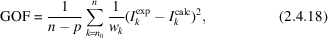where p is the number of parameters used in fitting, wk is a relative weight of the intensity value at the kth pixel, and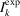and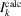are the experimentally measured and calculated intensity values for the kth pixel, respectively.

Structure models are described in parametric form (including experimental parameters, peak-shape parameters together with volume fractions of the phases and their fibre-textured components: p parameters altogether) and the p-dimensional parameter space is explored to calculate the GOF. The model with the smallest GOF is accepted. In phase analysis the best match is searched for by using the downhill simplex algorithm (Nelder & Mead, 1965). The semi-global simplex was found to be robust and allowed easy escape from local minima (Zuo & Spence, 1991) when used for fitting CBED patterns.

For polyphasic diffraction profiles, the volume fraction of phases is calculated at the end of the fitting procedure. It is assumed that the net diffraction intensity in each pixel is a linear combination of contributions of the individual phases (random and textured fractions are treated as independent model components). The over-determined set of equations is solved using least-squares minimization. The number of equations is reduced, while keeping the information content of all equations, by forming matrix A as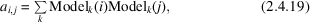where summation is performed for all pixels k for the model functions of the ith and jth phases, and vector b as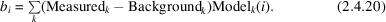The coefficients of the linear combination are obtained by solving for vector x the matrix equation Ax = b using matrix inversion.

The coefficients of this linear combination [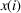] put the intensities of the peaks in phase i on the absolute scale. Imax(i), the intensity calculated on the absolute scale for the strongest (100%) diffraction peak of phase i, gives the intensity diffracted by one unit cell (structure factors are calculated for the atoms of one unit cell). Then x(i)/Imax(i) is the number of unit cells of phase i in the analysed volume. Consequently, the volume extended by phase i in the analysed volume is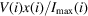, where V(i) is the volume of the unit cell of phase i. The volume fraction of phase fi is then given by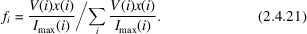In addition to volume fractions of phases and their fibre-textured components, the same method can determine the variation (contraction, dilation, distortions) of the unit cell, provided experimental parameters specific to electron diffraction (e.g. the camera length and pattern distortion) are properly calibrated. The reliability of the camera-length calibration (systematic error) is usually around 2% (Williams & Carter, 2009); in the best cases accuracy of better than 0.3% has been reported (Lábár et al., 2012). Consequently, only large variations in the lattice parameter can be determined reliably from powder electron diffraction data and the typical accuracy of powder X-ray diffraction cannot be attained.

There are two main advantages of phase analysis from powders by electron diffraction compared with X-ray diffraction. First, much smaller volumes can be studied. Diffraction information can be collected from thin layers of a few tens of nanometres thickness, enabling precise identification of the inspected volume. If needed, different lateral sections from different depths of a bulk sample can be studied by TEM, thus providing three-dimensional information about the sample. In a non-homogeneous sample, electron diffraction data can be collected from different areas, allowing detection of different phases or texture components at a spatial resolution and sensitivity superior to X-ray diffraction methods (Lábár et al., 2012).

The accuracy of the phase-content identification in a mixture for the major components is around 10–15% (Lábár et al., 2012). The detection limit depends on the scattering power of the component. A weakly scattering phase of Cr in a strongly scattering matrix of Ag could only be detected at the content of 2%, while the presence of 5% Ag in a relatively weakly scattering Ni matrix allowed full quantification of the two phases (Lábár et al., 2012). Thus, generally 5% (by volume) is accepted as the detection limit for powder electron diffraction experiments.

### References

Faber, J. & Fawcett, T. (2002). The Powder Diffraction File: present and future. Acta Cryst. B58, 325–332.Google Scholar
Gammer, C. C., Mangler, C., Rentenberger, C. & Karnthaler, H. P. (2010). Quantitative local profile analysis of nanomaterials by electron diffraction. Scr. Mater. 63, 312–315.Google Scholar
International Tables for Crystallography (2004). Volume C, Mathematical, Physical and Chemical Tables, edited by E. Prince. Dordrecht: Kluwer Academic Publishers.Google Scholar
Lábár, J. L. (2006). Phase identification by combining local composition from EDX with information from diffraction database. In Electron Crystallography, edited by T. E. Weirich, J. L. Lábár & X. Zou, Nato Science Series II, Vol. 211, pp. 207–218. Dordrecht: Springer.Google Scholar
Lábár, J. L. (2009). Electron diffraction based analysis of phase fractions and texture in nanocrystalline thin films, part II: implementation. Microsc. Microanal. 15, 20–29.Google Scholar
Lábár, J. L. & Adamik, M. (2001). ProcessDiffraction V1.2: new possibilities in manipulating electron diffraction ring patterns. Microsc. Microanal. 7 (Suppl. 2), 372–373.Google Scholar
Lábár, J. L. M., Adamik, M., Barna, B. P., Czigány, Z., Fogarassy, Z., Horváth, Z. E., Geszti, O., Misják, F., Morgiel, J., Radnóczi, G., Sáfrán, G., Székely, L. & Szüts, T. (2012). Electron diffraction based analysis of phase fractions and texture in nanocrystalline thin films, part III: application examples. Microsc. Microanal. 18, 406–420.Google Scholar
Moeck, P. & Fraundorf, P. (2007). Structural fingerprinting in the transmission electron microscope: overview and opportunities to implement enhanced strategies for nanocrystal identification. Z. Kristallogr. 222, 634–645.Google Scholar
Moeck, P. & Rouvimov, S. (2010). Precession electron diffraction and its advantages for structural fingerprinting in the transmission electron microscope. Z. Kristallogr. 225, 110–124.Google Scholar
Nelder, J. A. & Mead, R. (1965). A simplex method for function minimization. Comput. J. 7, 308–313.Google Scholar
Ungár, T., Gubicza, J., Ribárik, G. & Borbély, A. (2001). Crystallite size distribution and dislocation structure determined by diffraction profile analysis: principles and practical application to cubic and hexagonal crystals. J. Appl. Cryst. 34, 298–310.Google Scholar
Weickenmeier, A. & Kohl, H. (1991). Computation of absorptive form factors for high-energy electron diffraction. Acta Cryst. A47, 590–597.Google Scholar
Williams, D. B. & Carter, C. B. (2009). Transmission Electron Microscopy: a Textbook for Materials Science. New York: Springer.Google Scholar
Zuo, J. M. & Spence, J. C. H. (1991). Automated structure factor refinement from convergent-beam patterns. Ultramicroscopy, 35, 185–196.Google Scholar